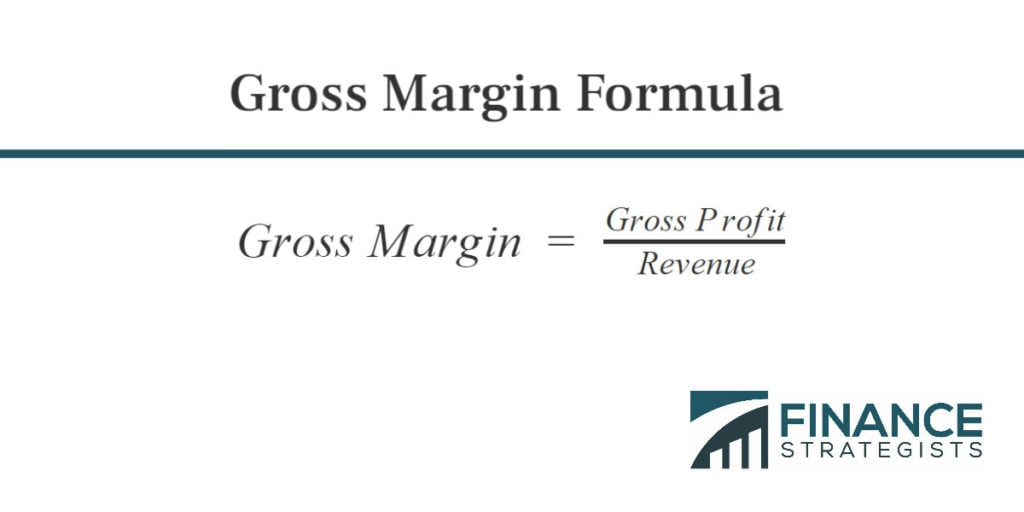# Gross Profit vs Gross Margin### Written byTrue Tamplin, BSc, CEPF® | Reviewed by Editorial Team

Updated on January 05, 2023

## Gross Profit vs Gross Margin

Although many people use the terms interchangeably, gross profit and gross margin are not the same.

Gross profit is a currency amount, while margin is a ratio or percentage.

Gross profit margin is the percentage left as gross profit after subtracting the cost of revenue from the revenue.

You calculate it by dividing the gross profit by the revenue.

## Gross Margin CalculationIn our coffee shop example above, the gross profit was \$80,000 from revenue of \$200,000.

This gives a gross profit margin of \$80,000 / \$200,000 = 40%.

## Gross Profit Difference

For every dollar in sales, the coffee shop has 40 cents in gross profit that it can use to pay for other business expenses (and hopefully have something left as net profit if it is a profitable business).

The easiest way to remember the difference between profit and margin is:

• sales/Income: Dollar amount (as in \$1,000)
• Margin: Percentage (as in 10%)

## A Higher Gross Profit Margin Is Better

If a company's gross margin increases, it means that the company is making more money per unit sold.

In other words, the company is becoming more efficient and generating more profits for the same amount of labor and material cost.

The best ways to increase gross margin are to raise prices or reduce the cost of producing the goods or services.

## Gross Profit vs Gross Margin FAQs

### What is gross profit?

Gross profit is the sum total of all income earned in a given year for an individual or a company.

### What is the difference between gross profit and gross margin?

Gross profit is a currency amount, while margin is a ratio or percentage. Gross profit margin is the percentage left as gross profit after subtracting the cost of revenue from the total revenue.

### How can gross margin be increased?

The best ways to increase gross margin are to raise prices or reduce the cost of producing the goods or services.

### What does it mean if gross margin increases?

If a company’s gross margin increases, it means that the company is making more money per unit sold.